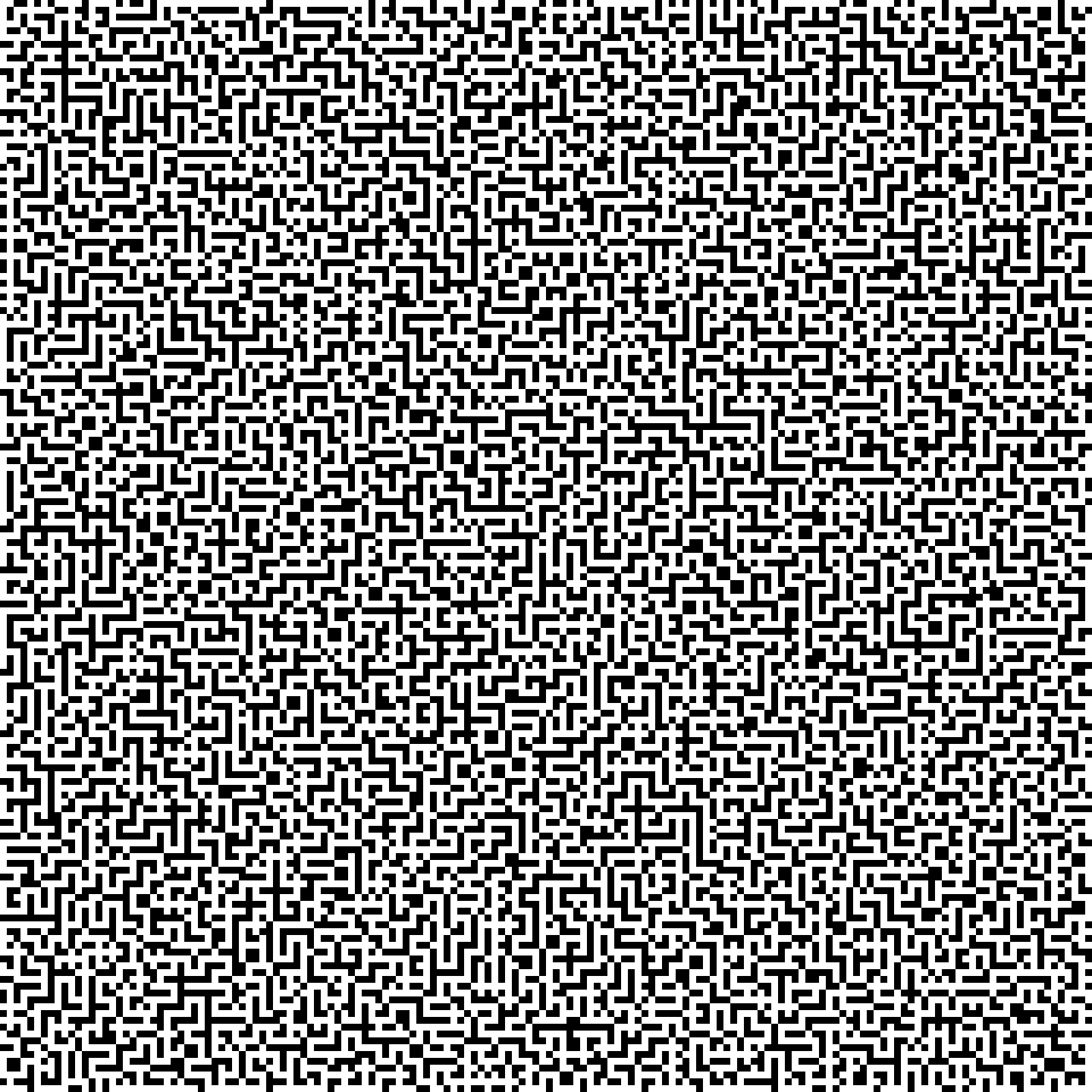### HackCon'18 - aMAZEing

ppc
tags: ctf challenge programming write-up
by pianka

In this write-up I’ll cover how I solved the aMAZEing programming challenge during HackCon’18.

We intercepted some weird transmission. Can you find what they are hiding?!

`nc 139.59.30.165 9300`

Since there is no downloadable binary, I’ll report every message from the server, as I’m sure it won’t be online forever.

We are welcomed by this text:

``````Get ready to solve some mazes.
We will send you a image over this socket. Give us the path which is required to go from position (0,0) in the top left to position (n, n) in the bottom right.
If no such path is possible please send back 'INVALID' without any quotes.

The path should be given in terms of WASD alphabets. Where they represent the folling:
W = Move up
A = Move left
S = Move down
D = Move right

``````

As soon as we press Enter we receive a binary image, ending with `Give us the path or write INVALID`. It’s time to set up a connection via pwntools and save that image to a file, so we can give it a look

``````from pwn import *
conn = remote('139.59.30.165', 9300)
while True:
line = conn.recvline(timeout=4)
if('Enter)' in line):
conn.recvline()
break
conn.send('\n')

ff = open('image.png', 'w+')
while True:
f = conn.recvline(timeout=4)
ff.write(bytearray(f))
if 'Give us the path or write INVALID' in f:
break;
ff.close()
``````

If we run this a bunch of time we’ll notice the server sends us different images, both in content and size. They all look sort of the same:As we can see it’s just a black-n-white maze, resembling a qr code. Each square is 10x10 pixels, so we can resize the big image to a tenth of the original size and save up a bit of space. Now it’s just a matter of solving the maze and sending the solution to the server. I could have written the solver on my own, but to save time for the competition I’ve decided to take the code from the Internet. I found this cool maze solver on GitHub which I had to adapt just to load my custom array and to give me the solution in terms of WASD-y keys.

To do that I added two methods to the class (I know the second one is a bit messy, but it works):

``````def read_personal(self, arr):
maze = []
for line in arr:
maze.append(list(line))
self.data = maze

def return_solution(self, sol='', where=None):
start_symbol = 'S'
end_symbol = 'E'
directions = (0, 1), (1, 0), (0, -1), (-1, 0)
direction_marks = '>', 'v', '<', '^'
direction_key = 'D', 'S', 'A', 'W'

where = where or self.find(start_symbol)
if self.get(where) == end_symbol:
# standing on the end cell
return sol

if self.get(where) == start_symbol:
if self.get(next_cell) in direction_marks and self.get(next_cell) != '<':
return self.return_solution('D', next_cell)
else:

if self.get(where) not in direction_marks:
return

direction = directions[direction_marks.index(self.get(where))]
return self.return_solution(sol + direction_key[directions.index(direction)], next_cell)
``````

Another adjustment I had to make was to increase the recursion limit

``````import sys

sys.setrecursionlimit(10000000)
``````

It is now quite straight-forward

``````from PIL import Image
import numpy
import maze as solver

# Resizing
im = Image.open('image.png')
im = im.resize((im.width / 10, im.height / 10), Image.NEAREST)
im_arr = numpy.array(im)
im.close()

# Converting pixel in the char array the solver wants :)
# Also adding walls all around the maze
maze = []
ll = ''
for i in range(0, im.height + 2):
ll += '#'
maze.append(ll)
for line in im_arr:
l = '#'
for pixel in line:
if pixel == 0:
l += '#'
else:
l += ' '
l += '#'
maze.append(l)
maze.append(ll)
maze = '#S' + maze[2:]
maze[im.height] = maze[im.height][:-2] + 'E#'

# Solving and getting the solution
maze_obj = solver.Maze()
solution = solver.solve(maze_obj)
if solution:
conn.send(maze_obj.return_solution() + '\n')
else:
conn.send('INVALID\n')
``````

This was perfect and had to be repeated a few times as the server continues sending us mazes to solve.

At the end, after a last `WooHoo you got it correct. Now solve a few more and get your flag.` which makes us think there is still a maze to solve and creates EOF errors when we are trying to read another image (I’ve spent like half an hour trying to solve that error grr), we get the flag:

``````Congratulations the flag is d4rk{1_h0p3_y0u_tr1ed_paint_lm40}c0de
``````

Generally speaking this challenge was not so hard to solve, but I wasted a lot of time debugging the solver and having troubles with the final communication with the server

Full crappy messy code on my GitHub